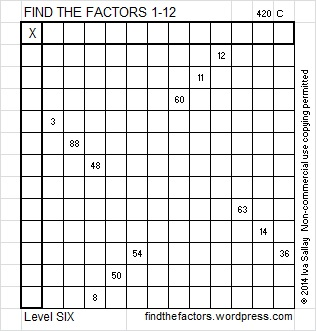127 and Level 6

• 127 is a prime number.
• Prime factorization: 127 is prime.
• The exponent of prime number 127 is 1. Adding 1 to that exponent we get (1 + 1) = 2. Therefore 127 has exactly 2 factors.
• Factors of 127: 1, 127
• Factor pairs: 127 = 1 x 127
• 127 has no square factors that allow its square root to be simplified. √127 ≈ 11.2694How do we know that 127 is a prime number? If 127 were not a prime number, then it would be divisible by at least one prime number less than or equal to √127 ≈ 11.3. Since 127 cannot be divided evenly by 2, 3, 5, 7, or 11, we know that 127 is a prime number.

Thinking process using divisibility tricks to prove that 127 is not divisible by 2, 3, 5, 7, or 11:

• 127 is not even, so 127 is not divisible by 2.
• 1 + 2 + 7 = 10 which is not divisible by 3, so 127 is not divisible by 3.
• The last digit of 127 is not 5 or 0, so 127 is not divisible by 5.
• The divisibility trick for 7 requires us to split 127 into 12 and 7. We double 7 and find the difference between the double and 12. We get (2 x 7) – 12  = 14 – 12 = 2. Since 2 is not divisible by 7, 127 is also not divisible by 7.
• To perform the divisibility trick for 11 we look at the digits another way: Digits that are in an odd position are in regular type and the digit in an even position is in bold type: 127. We find the sum of the numbers typed in regular type (1 + 7  = 8) and the sum of the number(s) typed in bold type (2) and subtract the smaller sum from the larger sum (8 – 2 = 6). If the difference were a multiple of 11, than the original number would also be a  multiple of 11 (and divisible by 11). Since 6 is not a multiple of 11, we know 127 is not divisible by 11.

Therefore the only factor pair that exists for 127 is 1 x 127, and it is a prime number.

127 is never a clue in the FIND THE FACTORS puzzles.

Spotting the logic needed to begin this Level 6 puzzle should be easier than usual. If you have completed Level 5 puzzles, but have never before attempted a Level 6 puzzle, try this one !Excel file of this week’s puzzles and last week’s factors: 12 Factors 2014-05-191.Steve Morris
2.ivasallay